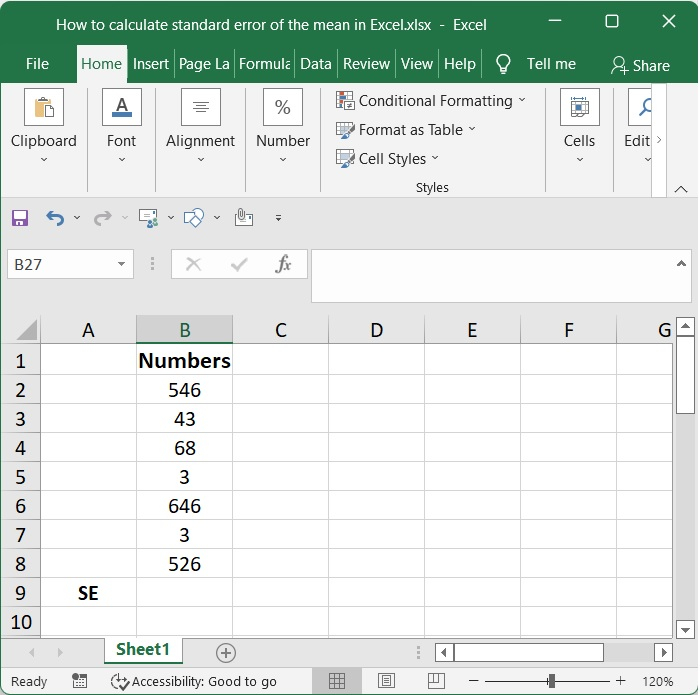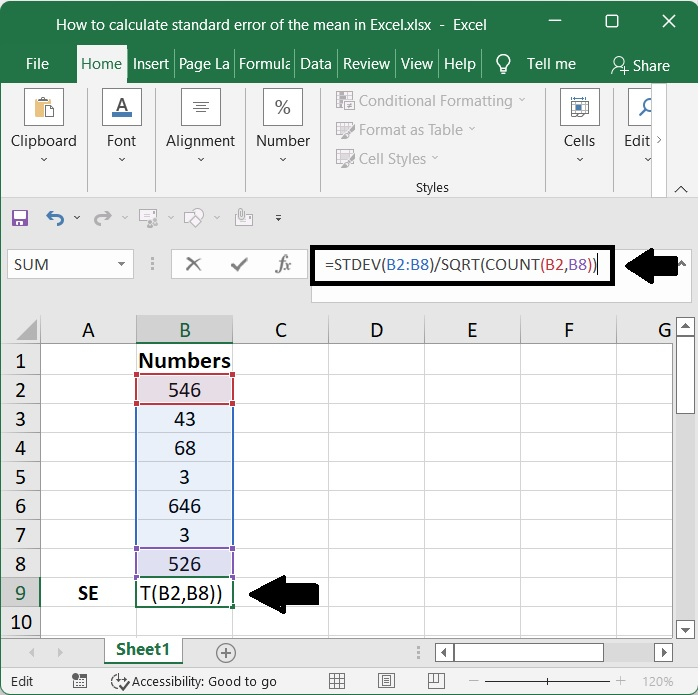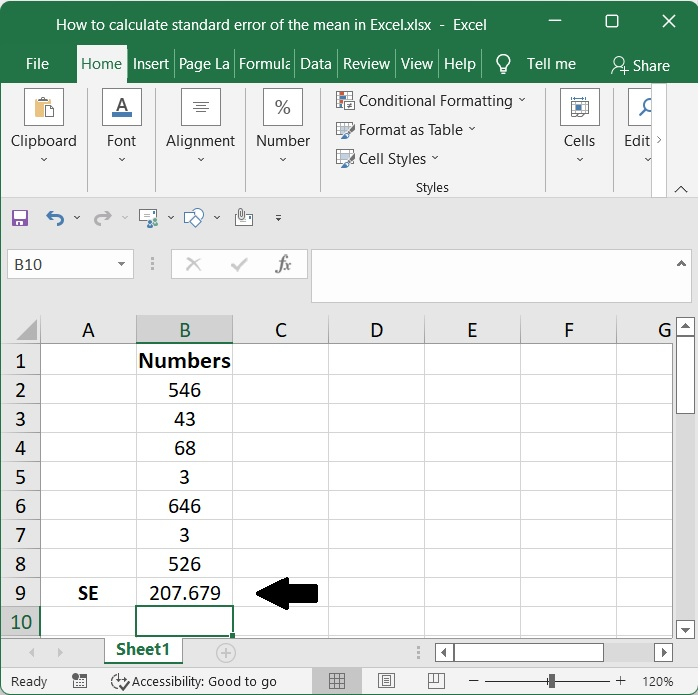# How to calculate standard error of the mean in Excel?

This same standard error would be a crucial statistic that serves as a parameter. But are you able to determine it throughout the process of sampling and distribution. A standard error of measurement, often known as the SEM, is indeed a statistical measurement which also provides an approximation of the difference between both the sample mean of the data and the actual population mean.

You are aware that the Standard Error is equal to the Standard Deviation divided by the Square Root of the Total Number of Samples; hence, we can convert this into an Excel formula by writing Standard Error = STDEV/SQRT (COUNT).

## Step 1

Excel provides us with the ability to do variance calculations to use both the mathematical equation as well as built-in algorithms including the STDEV.S and SQRT.

Consider the following scenario: the standard error of something like the mean is being sought for a sample collection of data that contains integer values.## Step 2

To show the standard error of the mean, go to the cell in which you wish it to appear.

You have the option of selecting the cell in which you want to insert the calculated result, followed by typing in the formula.

=STDEV(B2:B8)/SQRT(COUNT(B2:B8))


You will need to hit the Enter key in order to see the result.## Step 3

The standard error of the mean is now available for you to get, as seen in the image below## Conclusion

We were taught how to compute the standard error of the mean through the utilisation of a statistical model in its most condensed form as well as through the utilisation of functions also including STDEV.S and SQRT because there is no dedicated function available for the calculation of the standard error of the mean directly.